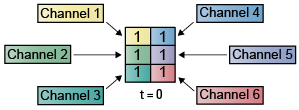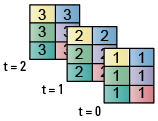## Deconstruct Multichannel Signals for Sample-Based Processing

In sample-based processing, blocks process signals one sample at a time. Each element of the input signal represents one sample in a distinct channel. For example, from a sample-based processing perspective, the following 3-by-2 matrix contains the first sample in each of six independent channels.When you configure a block to perform sample-based processing, the block interprets scalar input as a single-channel signal. Similarly, the block interprets an M-by-N matrix as multichannel signal with M*N independent channels. For example, in sample-based processing, blocks interpret the following sequence of 3-by-2 matrices as a six-channel signal.For more information about the recent changes to frame-based processing, see the Frame-based processing changes section of the DSP System Toolbox™ Release Notes.

### Split Multichannel Signals into Individual Signals

Multichannel signals, represented by matrices in the Simulink® environment, are frequently used in signal processing models for efficiency and compactness. Though most of the signal processing blocks can process multichannel signals, you may need to access just one channel or a particular range of samples in a multichannel signal. You can access individual channels of the multichannel signal by using the blocks in the Indexing library. This library includes the Selector, Submatrix, Variable Selector, Multiport Selector, and Submatrix blocks.

You can split a multichannel based signal into single-channel signals using the Multiport Selector block. This block allows you to select specific rows and/or columns and propagate the selection to a chosen output port. In this example, a three-channel signal of size `3`-by-`1` is deconstructed into three independent signals of sample period 1 second.

1. Open the Multiport Selector Example 1 model by typing `ex_splitmltichsbsigsind` at the MATLAB® command line.2. Double-click the Signal From Workspace block, and set the block parameters as follows:

• Signal = `randn(3,1,10)`

• Sample time = `1`

• Samples per frame = `1`

Based on these parameters, the Signal From Workspace block outputs a three-channel signal with a sample period of 1 second.

3. Save these parameters and close the dialog box by clicking .

4. Double-click the Multiport Selector block. Set the block parameters as follows, and then click :

• Select = `Rows`

• Indices to output = `{1,2,3}`

Based on these parameters, the Multiport Selector block extracts the rows of the input. The Indices to output parameter setting specifies that row 1 of the input should be reproduced at output 1, row 2 of the input should be reproduced at output 2, and row 3 of the input should be reproduced at output 3.

5. Run the model.

6. At the MATLAB command line, type `dsp_examples_yout`.

The following is a portion of what is displayed at the MATLAB command line. Because the input signal is random, your output might be different than the output show here.

```dsp_examples_yout(:,:,1) = -0.1199 dsp_examples_yout(:,:,2) = -0.5955 dsp_examples_yout(:,:,3) = -0.0793```

This signal is the first row of the input to the Multiport Selector block. You can view the other two input rows by typing `dsp_examples_yout1` and `dsp_examples_yout2`, respectively.

You have now successfully created three single-channel signals from a multichannel signal using a Multiport Selector block.

### Split Multichannel Signals into Several Multichannel Signals

Multichannel signals, represented by matrices in the Simulink environment, are frequently used in signal processing models for efficiency and compactness. Though most of the signal processing blocks can process multichannel signals, you may need to access just one channel or a particular range of samples in a multichannel signal. You can access individual channels of the multichannel signal by using the blocks in the Indexing library. This library includes the Selector, Submatrix, Variable Selector, Multiport Selector, and Submatrix blocks.

You can split a multichannel signal into other multichannel signals using the Submatrix (Simulink) block. The Submatrix block is the most versatile of the blocks in the Indexing library because it allows arbitrary channel selections. Therefore, you can extract a portion of a multichannel signal. In this example, you extract a six-channel signal from a 35-channel signal (a matrix of size 5-by-7). Each channel contains one sample.

1. Open the Submatrix Example model by typing `ex_splitmltichsbsigsev` at the MATLAB command line.2. Double-click the Constant block, and set the block parameters as follows:

• Constant value = `rand(5,7)`

• Interpret vector parameters as 1–D = Clear this check box

• Sample Time = `1`

Based on these parameters, the Constant block outputs a constant-valued signal.

3. Save these parameters and close the dialog box by clicking .

4. Double-click the Submatrix block. Set the block parameters as follows, and then click :

• Row span = ```Range of rows```

• Starting row = `Index`

• Starting row index = `3`

• Ending row = `Last`

• Column span = ```Range of columns```

• Starting column = ```Offset from last```

• Starting column offset = `1`

• Ending column = `Last`

Based on these parameters, the Submatrix block outputs rows three to five, the last row of the input signal. It also outputs the second to last column and the last column of the input signal.

5. Run the model.

The model should now look similar to the following figure.Notice that the output of the Submatrix block is equivalent to the matrix created by rows three through five and columns six through seven of the input matrix.

You have now successfully created a six-channel signal from a 35-channel signal using a Submatrix block. Each channel contains one sample.